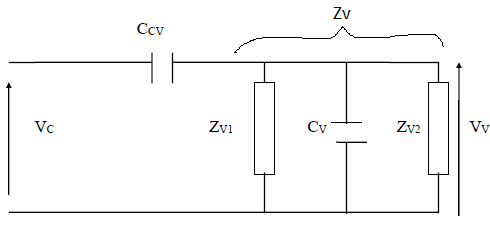# Solving voltage divider involving capacitors

wu_weidong

## Homework StatementGiven ZV1 = ZV2 = 100Ω,
ZCCV = ZCV = 2000/j = -2000j, and
VV/VC = 0.04789.

I'm trying to get the given VV/VC result.

## Homework Equations

I know that
1/ZV = 1/ZV1 + 1/ZV2 + 1/ZCV
VV = ZV / (ZV + ZCCV) * VC

## The Attempt at a Solution

1/ZV = 1/ZV1 + 1/ZV2 + 1/ZCV = 1/100 + 1/100 + j/2000
|1/ZV| = √(0.022 + (1/2000)2) = 0.02
ZV = 50Ω

VV/VC = ZV / (ZV + ZCCV) = 50 / (50 - 2000j)
VC/VV = (50 - 2000j) / 50 = 1 - 40j
|VC/VV| = √(12 + 402) = 40.01
VV/VC = 1/40.01 = 0.02499
which is about half of the value I should get.

Where did I go wrong?